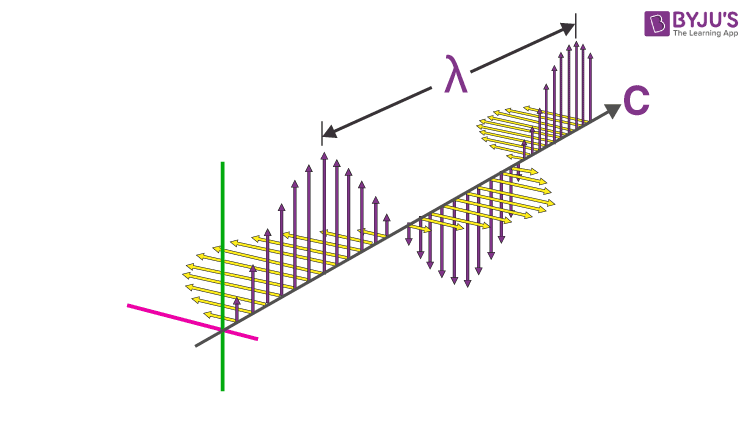# Properties of Electromagnetic Waves

Electromagnetic waves consist of two waves oscillating perpendicular to each other. One is the oscillating electric field and the other one is an oscillating magnetic field. The direction of propagation of the wave is perpendicular to the electric field and the magnetic field. They are transverse waves and do not require a material medium for propagation. They travel at the speed of light which is 3 x 108 m/s.## Basic Properties of Electromagnetic Waves

Below are some important properties :

Property 1: Electromagnetic waves are transverse in nature.

Property 2: They propagate by varying electric fields and magnetic fields, such that these two fields are at right angles to each other and at a right angle with the direction of propagation of the wave.

Property 3: Electromagnetic waves travel with a constant velocity in vacuum. The speed of the waves is 3 x 108 m/s.

Property 4:  Electromagnetic waves are non-mechanical waves. They do not require any material medium for propagation.

Property 5: They obey the equation c = fλ. Here, f is the frequency is Hertz and λ is the wavelength metres. The product of wavelength and frequency is equal to a constant c, the speed of light which is equal to 3 x 108 m/s. From this relation between wavelength, frequency and speed of light we can understand that the electromagnetic waves will travel with the speed of light regardless of wavelength and frequency.

Property 6: The oscillating electric field and magnetic field are in the same phase. The ratio of the amplitudes of the electric field and the magnetic field is equal to the velocity of the wave.

Test Your Knowledge On Properties Of Electromagnetic Waves!